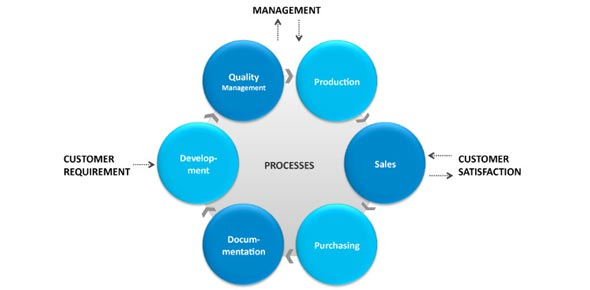# Componenet 10 Continued

17 Questions | Total Attempts: 137Settings• 1.
Which of the following best describes a process
• A.

A small batch of work

• B.

A series of activities that produce work

• C.

A group of people that undertake work

• D.

An organization that peforms work

• 2.
Process analysis includes which of the following
• A.

Knowledge of process components and relationships between them

• B.

Identification of opportunites for improvement

• C.

Both

• D.

Neither

• 3.
• A.

A change in a process

• B.

Anticipation of a good outcome

• C.

Both

• D.

Neither

• 4.
A worfkflow is a process
• A.

True

• B.

False

• 5.
Workflow analysis includes which of the following?
• A.

Knowledge of process components and relationships between them

• B.

Identification of opportunites for improvement

• C.

Both

• D.

Neither

• 6.
Which of the following is NOT considered knowledge?
• A.

True and justified beliefs

• B.

Declaritive facts

• C.

Procedural facts

• D.

Beliefs that may be true

• 7.
Which of the following is NOT one of the IOM characterisitics of quality heallthcare?
• A.

Efficient

• B.

Timely

• C.

Patient centered

• D.

Equitable

• 8.
Which of the following is NOT a characteristic of menaingful use of Health IT
• A.

Refers to the use of Health IT to improve patient care

• B.

Includes eprescribing and provider order entry

• C.

Has been in legislation for over 20 years

• D.

Includes incentives for meeting requiremnts for care

• 9.
Mark the symbols that are common flowchart symbols
• A.

Pentagon

• B.

Arrow

• C.

Oval

• D.

Dodecahedron

• 10.
What symbol should be used to diagram the following process step: "the patient is a new patient"
• A.

Pentagon

• B.

Diamond

• C.

Oval

• D.

Rectangle

• 11.
Which of the following should be used to diagram the following text" the new patient intake form is received, the information is confirmed with the patient then entered into the EMR"
• A.

Terminal symbol then two parallel process boxes

• B.

Circle, process box, then diamond

• C.

Document symbol, then two sequential process boxes

• D.

Document box, then a diamond followed by a terminal symbol

• 12.
An analyst needs to show the context in which a process operates, Which of the following methodologies is the most appropriate?
• A.

Yourdon

• B.

UML

• C.

Flowchart

• D.

Entity realtionship diagram

• 13.
An analyst needs to show information movement and the order of the steps which of the following diagram notations should be used?
• A.

Use case scenario

• B.

Stakeholder diagram

• C.

Context diagram

• D.

Data flow diagram

• 14.
An analyst needs to show information content. Which of the following notations would be appropriate?
• A.

Flowchart

• B.

E-R diagram

• C.

Dataflow diagram

• D.

Workflow diagram

• 15.
An Analyst needs to show detailed process steps. Which of the following diagrams is the most appropriate?
• A.

UML diagram

• B.

ISO data flow diagram

• C.

Context diagram

• D.

E-R diagram

• 16.
Indicate which statement is true:
• A.

Information flow and workflow are the same thing

• B.

Information flow and workflow can be shown on a context diagram

• C.

Information flow and process flow can be shown on an E-R diagram

• D.

Information flow and process flow can be shown on a flowchart

• 17.
Which of the following is a true statement
• A.

Multiple process aspects may be represented on some diagrams

• B.

There is only one type of diagram for each process aspect

• C.

There is not any overlap in the symbols used in different notations

• D.

There is only one correct representation for any process